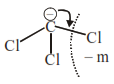# Arrange the carbanions,

Question:

Arrange the carbanions, $\left(\mathrm{CH}_{3}\right)_{3} \overline{\mathrm{C}}, \overline{\mathrm{C}} \mathrm{Cl}_{3},\left(\mathrm{CH}_{3}\right)_{2} \overline{\mathrm{CH}}, \mathrm{C}_{6} \mathrm{H}_{5} \overline{\mathrm{C}} \mathrm{H}_{2}$, in order of their decreasing stability

1. $\overline{\mathrm{CCl}_{3}}>\mathrm{C}_{6} \mathrm{H}_{5} \overline{\mathrm{C}} \mathrm{H}_{2}>\left(\mathrm{CH}_{3}\right)_{2} \overline{\mathrm{C}} \mathrm{H}>\left(\mathrm{CH}_{3}\right)_{3} \overline{\mathrm{C}}$

2. $\left(\mathrm{CH}_{3}\right)_{3} \overline{\mathrm{C}}>\left(\mathrm{CH}_{3}\right)_{2} \overline{\mathrm{C}}+\mathrm{C}_{6} \mathrm{H}_{5} \overline{\mathrm{C}}{ }_{2}>\overline{\mathrm{C}} \mathrm{Cl}_{3}$

3. $\mathrm{C}_{6} \mathrm{H}_{5} \overline{\mathrm{C}} \mathrm{H}_{2}>\overline{\mathrm{C}} \mathrm{Cl}_{3}>\left(\mathrm{CH}_{3}\right)_{3} \overline{\mathrm{C}}>\left(\mathrm{CH}_{3}\right)_{2} \overline{\mathrm{C}} \mathrm{H}$

4. $\left(\mathrm{CH}_{3}\right)_{2} \overline{\mathrm{C}} \mathrm{H}>\overline{\mathrm{C}} \mathrm{Cl}_{3}>\mathrm{C}_{6} \mathrm{H}_{5} \overline{\mathrm{CH}_{2}}>\left(\mathrm{CH}_{3}\right)_{3} \overline{\mathrm{C}}$

Correct Option: 1

Solution: